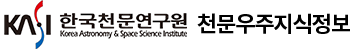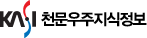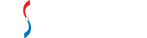통합검색

통합검색

인기검색어논문 상세보기

• 홈으로 이동
EVOLUTION OF ORBIT AND ROTATION OF A PSEUDO-SYNCHRONOUS BINARY SYSTEM ON THE MAIN SEQUENCE
• 저자
Li, Lin-Sen
• 저널명
The Korean Astronomical Society
• 발행년도
2018
• 권호
V .51, N .1
• 원문보기
We study the pseudo-synchronous orbital motion of a binary system on the main sequence. The equations of the pseudo-synchronous orbit are derived up to $O(e^4)$ where e is the eccentricy of the orbit. We integrate the equations to present their solutions. The theoretical results are applied to the evolution of the orbit and spin of the binary star Y Cygni, which has a current eccentricity of $e_0\;=\;0.142$ . We tabulate our numerical results for the evolution of the orbit and spin per century. The numerical results for the semi-major axes and rotational angular velocities in the evolutional time scales of three stages (synchronization, circularization, and collapse time scale) are also tabulated. Synchronization is achieved in about $5{\times}10^3\;years$ followed by circularization lasting about $1{\times}10^5\;years$ before decaying in $2{\times}10^5\;years$ .